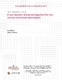## A non-heuristic distributed algorithm for non-convex constrained optimization2013-02-15
Matei, Ion
Baras, John
##### Abstract
In this paper we introduce a discrete-time, distributed optimization algorithm executed by a set of agents whose interactions are subject to a communication graph. The algorithm can be applied to optimization problems where the cost function is expressed as a sum of functions, and where each function is associated to an agent. In addition, the agents can have equality constraints as well. The algorithm can be applied to non-convex optimization problems with equality constraints, it is not consensus-based and it is not an heuristic. We demonstrate that the distributed algorithm results naturally from applying a first order method to solve the first order necessary conditions of an augmented optimization problem with equality constraints; optimization problem whose solution embeds the solution of our original problem. We show that, provided the agents’ initial values are sufficiently close to a local minimum, and the step-size is sufficiently small, under standard conditions on the cost and constraint functions, each agent converges to the local minimum at a linear rate.